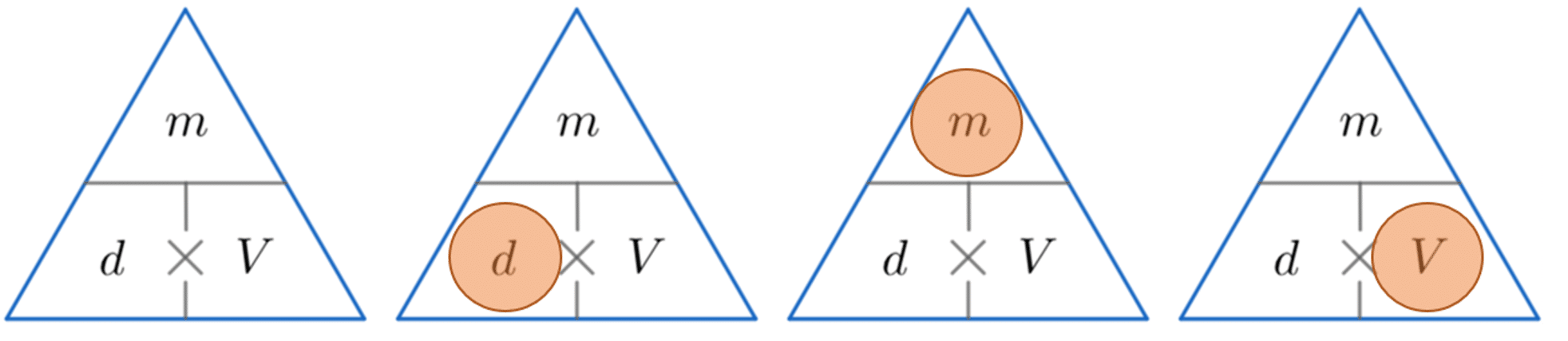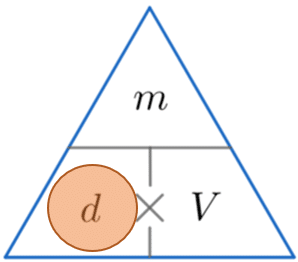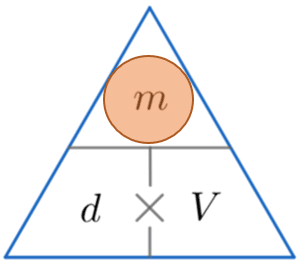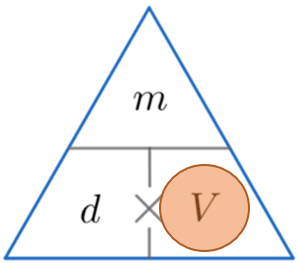# Density Mass Volume

GCSEKS3Level 4-5AQACambridge iGCSEEdexcelEdexcel iGCSEOCRWJEC

## Density Mass Volume

Density is a measure of how tightly the mass of an object is packed into the space it takes up. It can be calculated by dividing mass by volume.

Make sure you are happy with the following topics before continuing.

Level 4-5GCSEKS3AQAEdexcelOCRWJECCambridge iGCSEEdexcel iGCSE

## Density Mass Volume – Formula

Density, mass and volume are all related by the formula,

$d = \dfrac{m}{V}$

where $d$ is density, $V$ is volume, and $m$ is mass. This can be rearranged in order to find volume or mass depending on which quantities you are given and what the question asks you to find.

Mass is normally measured in grams, g, or kilograms, kg, whereas, volume is normally measured in cubic centimetres, cm$^3$, or cubic metres, m$^3$.

This means when dividing mass by volume, the resulting units are typically grams per cubic centimetre (g/cm$^3$), or kilograms per cubic metre (kg/m$^3$). These are compound units (for more information, see conversions revision).

Level 4-5GCSEKS3AQAEdexcelOCRWJECCambridge iGCSEEdexcel iGCSE

## Density Mass Volume – Formula Triangle

A handy way of remembering how to calculate either density, mass or volume is to use the triangles below.

The horizontal line means divide and the $\times$ symbol means multiply.

We then cover up the one we want to find (represented by a red circle) and carry out the calculation with the other two values from the triangle.Level 4-5GCSEKS3AQAEdexcelOCRWJECCambridge iGCSEEdexcel iGCSE

## Example 1: Calculating Density

An object has a mass of $570$ g and a volume of $2280$ cm$^3$. Calculate its density.

[2 marks]

We’re looking for density, so constructing the triangle and covering $d$, we see we must divide the mass by the volume. So

$\text{density } = \dfrac{570}{2280} = 0.25$ g/cm$^3$Level 4-5GCSEKS3AQAEdexcelOCRWJECCambridge iGCSEEdexcel iGCSE

## Example 2: Calculating Mass

A cat has volume $0.004$ m$^3$ and density $980$ kg/m$^3$. Calculate the mass of the cat.

[2 marks]

We’re looking for mass, so constructing the triangle and covering $m$, we see that to calculate the mass we must multiply the density by the volume. So

$\text{ Mass } = 980 \times 0.004 = 3.92$ kgLevel 4-5GCSEKS3AQAEdexcelOCRWJECCambridge iGCSEEdexcel iGCSE

## Example 3: Calculating Volume

A bottle of water has a density of $1000$ kg/m$^3$ and mass of $0.5$ kg. Calculate the volume of the water bottle giving your answer in litres.

[2 marks]

We’re looking for volume, so covering $V$, we must divide the mass by the density. So

$\text{ Volume } = \dfrac{0.5}{1000} = 0.0005$ m$^3$

Multiplying this by $1000$ to convert into litres, gives us the final answer,

$\text{ Volume } = 0.5$ LLevel 4-5GCSEKS3AQAEdexcelOCRWJECCambridge iGCSEEdexcel iGCSE

## Density Mass Volume Example QuestionsWe are calculating the volume, so by covering up the $V$ we can see from the triangle above that we have to divide $m$ by $d$.

Before we can do this, however, we have to make sure that we have the correct units. The mass is in kilograms, but the density is in grams per cubic centimetre. This means that we have to first convert the kilograms into grams before proceeding.

$2$ kg $= 2000$ g

Therefore, the volume of the olive oil can be calculated as follows:

$\text{Volume} = 2000 \div 0.925 = 2162$cm$^3$Gold Standard EducationWe are calculating the  mass, so by covering up the $m$ we can see from the triangle above that we have to multiply $d$ by $V$.

However, we don’t know the volume, but we do know that the shape is a cube with a side length of $7$ m, so the volume of the cube is:

$7 \times 7 \times 7 = 343$ m$^3$

Now that we know the volume, we can multiply it by the density in order to calculate the mass:

$\text{Mass } = 343 \times 10,800,000 = 3,704,400,000$ kgGold Standard Education

To calculate the answer here, we need to recall the formula:

$\text{ density} = \text{ mass} \div \text{ volume}$

In this question, the mass is $2460$kg and the volume is $1.2$ m$^3$, so we simply need to substitute these values into the formula as follows:

$\text{ Density} = 2460$ kg $\div \, 1.2$ m$^3 = 2050$ kg/m$^3$Gold Standard Education

a) In order to calculate the overall volume of the block, we need to add the volume of metal $A$ and the volume of metal $B$.  Although we don’t have the volume of either metal, we have been given their masses and densities, so we can calculate the volume of each metal accordingly.

By rearranging the density formula, or using the triangle, we can work out how to calculate the volume:

$\text{density} = \text{ mass}\div \text{ volume}$

So:

$\text{volume} = \text{ mass}\div \text{ density}$

The volume of metal $A$ can be calculated as follows:

$1200$ g $\div \, 5$ g/cm$^3 = 240$ cm$^3$

The volume of metal $B$ can be calculated as follows:

$600$ g $\div \, 3$ g/cm$^3 = 200$ cm$^3$

Therefore, if metal $A$ has a volume of $240$ cm$^3$ and $B$ has a volume of $200$ cm$^3$, then their combined volume is simply:

$240$ cm$^3 + 200$ cm$^3 = 440$ cm$^3$

b) As we know from question a), the newly-formed block has a volume of $440$ cm$^3$.

We know that the mass of metal $A$ was $1200$ g and the mass of metal $B$ was $600$ g, so mass of the block is:

$1200$ g $+ \, 600$ g $= 1800$ g

The density of this block can be calculated by dividing the mass by the volume as follows:

$1800$ g $\div \, 440$ cm$^3 = 4.09$ g/cm$^3$Gold Standard Education

This is quite a challenging question with a lot of calculations going on.

Since we have been given the mass of metal $C$ and the ratio of metal $A$ and metal $B$ in metal $C$, we can therefore calculate the mass of metal $A$ and metal $B$.  If the ratio of metal $A$ to metal $B$ is $3 : 7$, that means that $\frac{3}{10}$ of the mass of metal $C$ comes from metal $A$ and the remaining $\frac{7}{10}$ is metal $B$.  (We are dealing in tenths here since the sum of the ratio is $10$.)

The mass of metal $A$ can be calculated as follows:

$2500$ g $\times \dfrac{3}{10} = 750$ g

The mass of metal $B$ can be calculated as follows:

$2500$ g $\times \dfrac{7}{10} = 1750$ g

We now know both the mass and density of both metals $A$ and $B$, meaning we can work out their respective volumes.

Since

$\text{density} = \text{ mass} \div \text {volume}$

then

$\text{volume} = \text{ mass} \div \text {density}$

The volume of metal $A$ can be calculated as follows:

$750$ g $\div \, 3.2$ g/cm$^3 = 234.375$ cm$^3$

The volume of metal $A$ can be calculated as follows:

$1750$ g $\div \, 5.5$ g/cm$^3 = 318.18$ cm$^3$

If metal $A$ has a volume of $234.375$ cm$^3$ and metal $B$ has a volume of $318.18$ cm$^3$, then their combined volume is the volume of metal $C$.

Volume of metal $C$ = $234.375 + 318.18 = 552.5568$ cm$^3$

We now know both the mass and volume of metal $C$, so we are now able to calculate its density.

Density of metal $C$ = $2500$ g $\div \, 552.5568$ cm$^3 = 4.5$ g/cm$^3$Gold Standard Education

## Density Mass Volume Worksheet and Example Questions

### (NEW) Density Mass Volume Exam Style Questions - MME

Level 4-5GCSENewOfficial MME

## Density Mass Volume Drill Questions

Level 4-5GCSE

### Speed and Density - Drill Questions

Level 4-5GCSEGold Standard Education

£19.99 /month. Cancel anytime

Level 6-7GCSEKS3

Level 4-5GCSEKS3